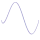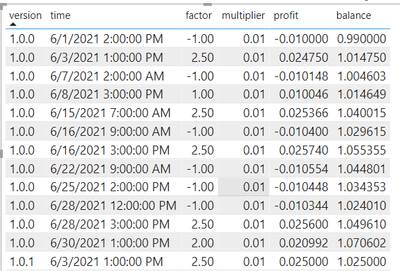cancel
Showing results for
Did you mean:Frequent Visitor

## How can I create these calculated measures?

Good day, I have difficulty creating these measures and hope to receive help 🙂 This is what I am trying to create:Profit formula is: factor * multiplier * previous row balance
Balance formula is: profit + previous row balance

Example:

Row 2 profit: 2.5 * 0.01 * 0.99 (or 99%) = 2.48%

Row 2 balance: 2.48% + 99% = 101.48

As there is no previous balance value for the first row, the calculation for row 1 is different!
Row 1 profit: factor * multiplier * 1

Row 1 balance: profit + 1

How can I create these measures? Thank you in advance for taking time to do this! Cheers! 😁

1 ACCEPTED SOLUTIONCommunity Support

Hi @greendate ,

This is a bit like a cumulative multiplication algorithm. power bi only supports the calculation of existing data, and cannot perform the next calculation based on its own calculation results. But we can optimize this algorithm.
Here is the logic of my calculation, you can refer to it.

assum a = factor * multiplier

p2 = b1 + a1

p3 = b2 + a2

p4 = b3 + a3

and

b2 = p2+b1

b3 = p3+b2

b4 = p4+b3

so ->

b2 = b1(1+a2)

b3 = b1(1+a2)*(1+a3)

b4 = b1(1+a2)*(1+a3)*(1+a4)

And the measures for [balance] and [profit] is:

``````balance =
VAR _b_s =
SUMMARIZE (
CALCULATETABLE (
report,
FILTER (
ALLEXCEPT ( report, 'report'[version] ),
[time] <= MAX ( 'report'[time] )
)
),
[version],
[time],
"fac",
[multiplier] * SUM ( report[factor] ) + 1
)
RETURN
PRODUCTX ( _b_s, [fac] )

profit =
VAR _factor =
SUM ( report[factor] )
VAR _a = [multiplier] * _factor
VAR _b_s =
SUMMARIZE (
CALCULATETABLE (
report,
FILTER (
ALLEXCEPT ( report, 'report'[version] ),
[time] < MAX ( 'report'[time] )
)
),
[version],
[time],
"fac",
[multiplier] * SUM ( report[factor] ) + 1
)
VAR _result =
PRODUCTX ( _b_s, [fac] ) * _a
RETURN
IF ( ISBLANK ( _result ), _a, _result )

``````

Resutl:Pbix in the end you can refer.

Best Regards

Community Support Team _ chenwu zhu

If this post helps, then please consider Accept it as the solution to help the other members find it more quickly.

3 REPLIES 3Community Support

Hi @greendate ,

This is a bit like a cumulative multiplication algorithm. power bi only supports the calculation of existing data, and cannot perform the next calculation based on its own calculation results. But we can optimize this algorithm.
Here is the logic of my calculation, you can refer to it.

assum a = factor * multiplier

p2 = b1 + a1

p3 = b2 + a2

p4 = b3 + a3

and

b2 = p2+b1

b3 = p3+b2

b4 = p4+b3

so ->

b2 = b1(1+a2)

b3 = b1(1+a2)*(1+a3)

b4 = b1(1+a2)*(1+a3)*(1+a4)

And the measures for [balance] and [profit] is:

``````balance =
VAR _b_s =
SUMMARIZE (
CALCULATETABLE (
report,
FILTER (
ALLEXCEPT ( report, 'report'[version] ),
[time] <= MAX ( 'report'[time] )
)
),
[version],
[time],
"fac",
[multiplier] * SUM ( report[factor] ) + 1
)
RETURN
PRODUCTX ( _b_s, [fac] )

profit =
VAR _factor =
SUM ( report[factor] )
VAR _a = [multiplier] * _factor
VAR _b_s =
SUMMARIZE (
CALCULATETABLE (
report,
FILTER (
ALLEXCEPT ( report, 'report'[version] ),
[time] < MAX ( 'report'[time] )
)
),
[version],
[time],
"fac",
[multiplier] * SUM ( report[factor] ) + 1
)
VAR _result =
PRODUCTX ( _b_s, [fac] ) * _a
RETURN
IF ( ISBLANK ( _result ), _a, _result )

``````

Resutl:Pbix in the end you can refer.

Best Regards

Community Support Team _ chenwu zhu

If this post helps, then please consider Accept it as the solution to help the other members find it more quickly.Frequent Visitor

Yes! This is what I was looking for. Thank you for helping @v-chenwuz-msft 😁 I have 1 more issue that I couldn't figure out yet. I want the sum of profit for total but it is not adding up correctly. At the moment I managed to get the desired output only by creating additional measure:``````new measure =
SUMX(
VALUES(report[time]),
report[profit])``````

How can I get the correct total sum for profit without creating another measure? Thank you in advance for helping! 😁

https://www.dropbox.com/s/q0qs0yhydc9fbk6/20220518-powerbi.pbix?dl=0Super User

You could use power query to add the previous balance to the current row, that would make the calculations much simpler and more efficient.

In power query, sort the data by the appropriate column, then add an index column, 0-based. You can then add a new custom column called previous_balance using something like

``if [index] = 0 then null else #”Added Index”[balance]{[index] – 1}``